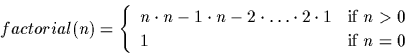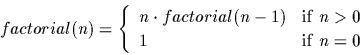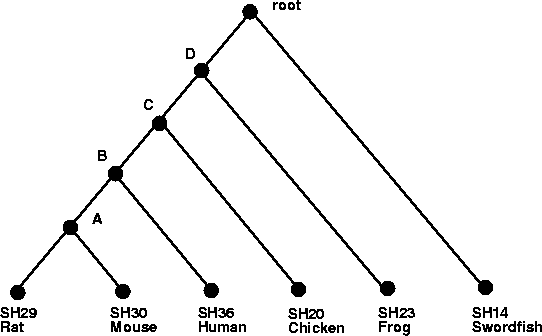Next: Input/Output Up: Iteration and Recursion Previous: The while Statement

# Recursive Calls

When a routine calls itself either directly or indirectly, it is said to be making a recursive call. Recursion is the third technique for repeatedly executing a command sequence. It, however, does not require any additional built-in Darwin commands. The basic idea behind solving problems via recursion is to break the instance of the problem into smaller and smaller pieces until the pieces are so small they can be solved trivially. We can view a recursive routine as consisting of two parts.

• The recursive case. When the instance of the problem is still too large to solve directly, we split the problem into two or more smaller parts. It must be the case that the entire problem can be solved if each of the subproblems are solved.
• The basis (or ground) case. When the instance of the problem is small enough to be solved directly, we solve it. If the routine is a function, return the result of this subproblem to the function which called it.

A necessary condition for recursion to work correctly is that progress must always be made at each invocation of the routine. If no progress is made, then the recursion will continue to dive infinitely deeper (and you will eventually run out of memory).

It is required by law that every programming language manual use the factorial function in an example. We give ours here. Recall the definition of the factorial of a positive integer:We could re-express this function in a more recursive style. This will help with the transition from the mathematical definition to the Darwin routine.Here the basis case of the function is when n=0. We simply return 1. The recursive case splits the problem into two cases n and factorial( n-1 ). It is not hard to see that multiplying n with the result of the subproblem factorial( n-1 ) will solve our original problem. The basis and recursive cases in our Darwin function correspond directly to the basis and recursive cases in the above equation.
> factorial := proc ( n )
>   if (n=0) then
>     return(1);
>   else
>     return(n * factorial(n-1));
>   fi;
> end:
>
> factorial(0);
1
> factorial(1);
1
> factorial(5);
120
> factorial(100);
9.3326215443944102e+157


Attempting to pass too large an integer to factorial may produce a rather extreme reaction from Darwin and the operating system you are working with. This is because the depth of recursion (the number of recursive calls) becomes so large, the system runs out of memory.6.1

Recursion is especially convenient for working with phylogenetic trees. Although there are many different variants of phylogenetic trees, the basic idea behind all of them is to represent the ancestral relationships between a set of taxa. We take this opportunity to introduce the Darwin data types Tree and Leaf as we will be working with them extensively in Chapter- Phylogenetic Trees of Part. We can represent a phylogenetic tree for a set of species as a complete binary tree6.2 with the vertices of the tree (the points or nodes) representing species and the edges (lines that connect points) representing ancestral relationships.There are two types of vertices:

• The leaves. These vertices have only one edge incident with them. When used to model phylogenetic trees, leaves typically represent extant species.
• The internal vertices. Complete binary trees come in two flavors: rooted and unrooted. In an unrooted complete binary tree, every internal vertex has two children and one parent. In a rooted complete binary tree, every internal vertex has two children and one parent except for one distinguished vertex which does not have a parent. This sole vertex is referred to as the root. When used to model phylogenetic trees, the internal vertices typically correspond to extinct species.

These trees capture the biologic notion of speciation events. In Figure, a speciation event occured in the ancestor of all fish (represented by the Swordfish X. Helleri) and the ancestor of amphibians and mammals. Next, a speciation occurred in the ancestor of the amphibians (represented by the frog here) and the ancestor of mammals and the chicken. Then comes the speciation between the ancestor of mammals and chicken, followed by a speciation of humans and the ancestor of mice and rats and, lastly, the divergence of mice and rats.

In Darwin we can define tree structures using the types Tree and Leaf. A Tree structure consists of a left child, a label and a right child. The left and right children consist of either another Tree structure or a Leaf structure. A Leaf structure contains only a label. The following code creates a Darwin tree for the tree shown in Figure.

> l_l_l_l := Tree( Leaf( 'SH30' ), 'A', Leaf( 'SH29' ) ):
> l_l_l := Tree( l_l_l_l, 'B', Leaf( 'SH36' ) ):
> l_l := Tree( l_l_l, 'C', Leaf( 'SH20' ) ):
> l := Tree( l_l, 'D',  Leaf( 'SH23' ) ):
> root := Tree( l, 'root' , Leaf( 'SH14' ) ):


This code can be found in file Samples/tree or from the COMPUTATIONAL BIOCHEMISTRY RESEARCH GROUP web site . This tree is a phylogenetic tree formed in Darwin for a set of sequences containing the SH2 domain. We will be using the SH1, SH2 and SH3 domains repeatedly throughout the latter chapters of this book. The following list (also contained in Samples/tree) maps labels for the sequences to the name of the organism from which they came.

> SH2toOrganism := [['SH20', 'CHICKEN'],  ['SH30', 'MOUSE'],
>    ['SH36', 'HUMAN'],  ['SH29', 'RAT'],  ['SH14','SWORDFISH'],
>    ['SH23','FROG']]:

Let us first design a recursive procedure to print out the tree in a more readable format.
  FindOrganism:= proc(label : string)
global SH2toOrganism;

for i from 1 to length(SH2toOrganism) do
current := SH2toOrganism[i];
if (current=label) then
return(current);
fi;
od;
return('Species not know');
end;

PrintTree := proc (T : {Leaf, Tree} )
# The basis case: T is a leaf
if (type(T, Leaf)) then            #   print out the organism name
printf('%s', FindOrganism(T));  #   corresponding to the label
else
printf(' [ ');                   # The recursive case: T is a
PrintTree(T['Left']);            #   tree with a left and right
printf(' ');                     #   child.  Recurse on both.
PrintTree(T['Right']);
printf(' ] ');
fi;
end;

[  [  [  [  [ MOUSE RAT ]  HUMAN ]  CHICKEN ]  FROG ]  SWORDFISH ]


The recursive case in PrintTree occurs when T is a Tree structure. Since phylogenetic trees are complete binary trees, T must have both a left and right subtree. We call PrintTree with the left subtree as a parameter and then we call PrintTree with the right subtree as a parameter. The basis case in our recursive procedure PrintTree occurs when the parameter T consists of only a Leaf structure. In this case it passes the label of the Leaf to the FindOrganism function. This function matches the Leaf label to the corresponding organism name found in SH2toOrganism and returns it to PrintTree.

We will see many more examples of recursion with Darwin trees in later chapters. It can be difficult to follow (never mind write) a recursive routine at first but the effort is worthwhile. What may take many lines of code to do iteratively, may take only a few lines recursively. In this way, recursion can offer a crisp elegant perspective of many routines.Next: Input/Output Up: Iteration and Recursion Previous: The while Statement
Gaston Gonnet
1998-09-15